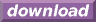#Calcute: freeware calculator

## Hyperbolic Arcsine (hyperbolic arc-sine, hyperbolic inverse sine, asinh)

The hyperbolic arc-sine is the inverse of the hyperbolic sine function. Calcute implements it with the asinh function. The inverse hyperbolic sine of a value x is the value y for which the hyperbolic sine of y is x. In other words if y = asinh(x) then x = sinh(y).

```asinh(1)
0.88137358702
```

In some mathematical texts and hand-held calculators, the notation sinh-1 is sometimes used to represent the hyperbolic arc-sine function. This is an unfortunate notational choice since it could also stand for the multiplicative inverse 1/sinh, which is a different function.

This function is sometimes called the "area hyperbolic sine". Another abbreviation sometimes used to represent it is "arcsinh".